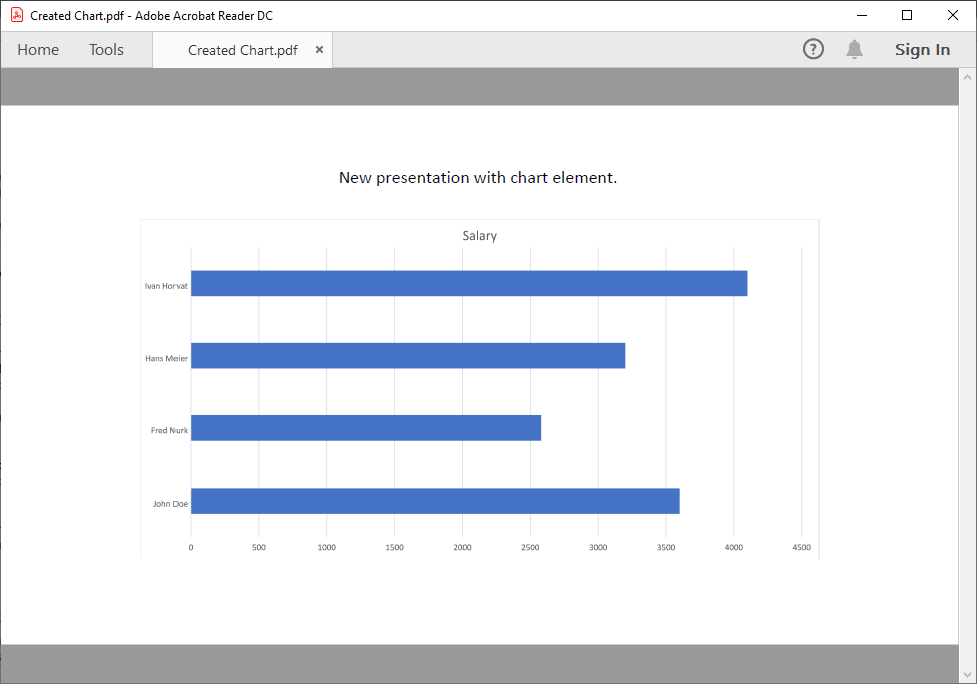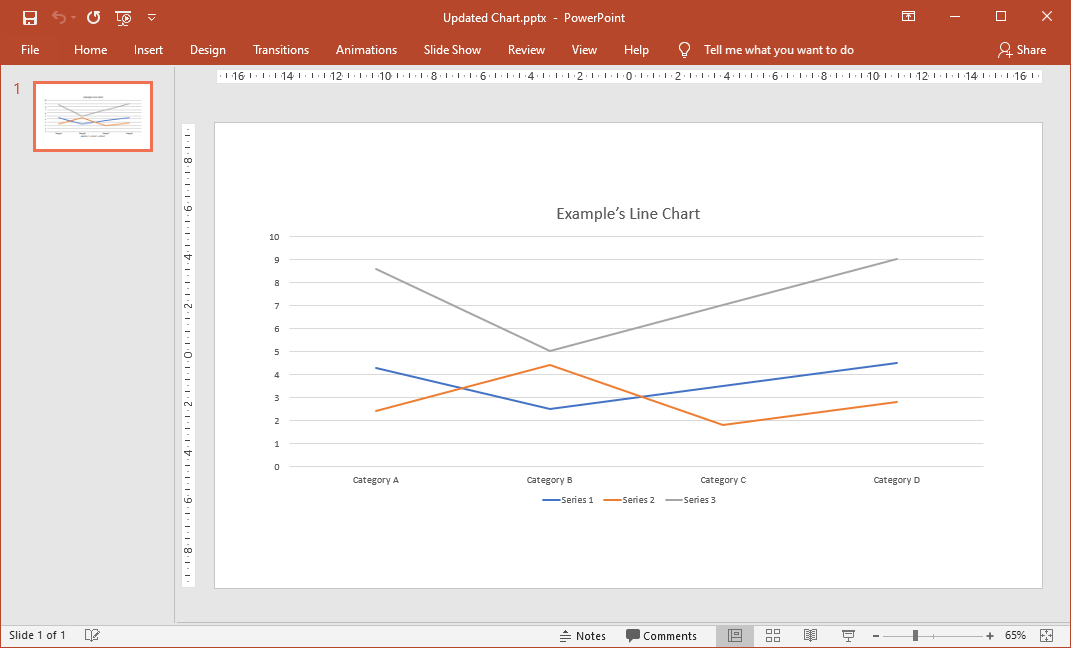# PowerPoint Charts in C# and VB.NET

`Chart` elements are drawings used for graphical data representation. GemBox.Presentation provides a support for working with charts with a help of GemBox.Spreadsheet component.

• With GemBox.Spreadsheet, GemBox.Presentation is able to create and update charts in PowerPoint presentations. It's also able to export presentations with charts to PDF, XPS or image format.
• Without GemBox.Spreadsheet, GemBox.Presentation is only able to preserve charts in PowerPoint presentations.

## Create chart

To enable GemBox.Presentation's chart support and work with `Chart` objects, you'll need to add a reference to GemBox.Spreadsheet library and call its `SpreadsheetInfo.SetLicense` method.

Note, if you don't have a GemBox.Spreadsheet license you can use its Free mode.

The following example shows how you can create a presentation with a chart element.Screenshot of PDF document with chart
``````using GemBox.Presentation;

class Program
{
static void Main()
{
// If using Professional version, put your GemBox.Presentation serial key below.

// If using Professional version, put your GemBox.Spreadsheet serial key below.

var presentation = new PresentationDocument();

// Add new PowerPoint presentation slide.
var slide = presentation.Slides.AddNew(SlideLayoutType.Custom);

// Add simple PowerPoint presentation title.
var textBox = slide.Content.AddTextBox(ShapeGeometryType.Rectangle,
116.8, 20, 105, 10, GemBox.Presentation.LengthUnit.Millimeter);

// Create PowerPoint chart and add it to slide.
var chart = slide.Content.AddChart(GemBox.Presentation.ChartType.Bar,
49.3, 40, 240, 120, GemBox.Presentation.LengthUnit.Millimeter);

// Get underlying Excel chart.
ExcelChart excelChart = (ExcelChart)chart.ExcelChart;
ExcelWorksheet worksheet = excelChart.Worksheet;

// Add data for Excel chart.
worksheet.Cells["A1"].Value = "Name";
worksheet.Cells["A2"].Value = "John Doe";
worksheet.Cells["A3"].Value = "Fred Nurk";
worksheet.Cells["A4"].Value = "Hans Meier";
worksheet.Cells["A5"].Value = "Ivan Horvat";

worksheet.Cells["B1"].Value = "Salary";
worksheet.Cells["B2"].Value = 3600;
worksheet.Cells["B3"].Value = 2580;
worksheet.Cells["B4"].Value = 3200;
worksheet.Cells["B5"].Value = 4100;

// Select data.
excelChart.SelectData(worksheet.Cells.GetSubrange("A1:B5"), true);

presentation.Save("Created Chart.%OutputFileType%");
}
}
``````
``````Imports GemBox.Presentation

Module Program

Sub Main()

' If using Professional version, put your GemBox.Presentation serial key below.

' If using Professional version, put your GemBox.Spreadsheet serial key below.

Dim presentation As New PresentationDocument()

' Add new PowerPoint presentation slide.
Dim slide = presentation.Slides.AddNew(SlideLayoutType.Custom)

' Add simple PowerPoint presentation title.
Dim textBox = slide.Content.AddTextBox(ShapeGeometryType.Rectangle,
116.8, 20, 105, 10, GemBox.Presentation.LengthUnit.Millimeter)

' Create PowerPoint chart and add it to slide.
Dim chart = slide.Content.AddChart(GemBox.Presentation.ChartType.Bar,
49.3, 40, 240, 120, GemBox.Presentation.LengthUnit.Millimeter)

' Get underlying Excel chart.
Dim excelChart As ExcelChart = DirectCast(chart.ExcelChart, ExcelChart)
Dim worksheet As ExcelWorksheet = excelChart.Worksheet

' Add data for Excel chart.
worksheet.Cells("A1").Value = "Name"
worksheet.Cells("A2").Value = "John Doe"
worksheet.Cells("A3").Value = "Fred Nurk"
worksheet.Cells("A4").Value = "Hans Meier"
worksheet.Cells("A5").Value = "Ivan Horvat"

worksheet.Cells("B1").Value = "Salary"
worksheet.Cells("B2").Value = 3600
worksheet.Cells("B3").Value = 2580
worksheet.Cells("B4").Value = 3200
worksheet.Cells("B5").Value = 4100

' Select data.
excelChart.SelectData(worksheet.Cells.GetSubrange("A1:B5"), True)

presentation.Save("Created Chart.%OutputFileType%")

End Sub

End Module
``````

For more information about charts, visit the Chart Components and Chart Formatting examples.

## Update chart

The following example shows how you can update chart element's data in a PowerPoint presentation.Screenshot of PowerPoint presentation with updated chart
``````using System.Linq;
using GemBox.Presentation;

class Program
{
static void Main()
{
// If using Professional version, put your GemBox.Presentation serial key below.

// If using Professional version, put your GemBox.Spreadsheet serial key below.

// Load input file and save it in selected output format
var presentation = PresentationDocument.Load("%#Chart.pptx%");

// Get PowerPoint chart.
var chart = ((GraphicFrame)presentation.Slides.Content.Drawings).Chart;

// Get underlying Excel chart and cast it as LineChart.
var lineChart = (LineChart)chart.ExcelChart;

// Add new line series which has doubled values from the first series.
.Values.Cast<double>().Select(val => val * 2));

presentation.Save("Updated Chart.%OutputFileType%");
}
}
``````
``````Imports System.Linq
Imports GemBox.Presentation

Module Program

Sub Main()

' If using Professional version, put your GemBox.Presentation serial key below.

' If using Professional version, put your GemBox.Spreadsheet serial key below.

' Load input file and save it in selected output format
Dim presentation = PresentationDocument.Load("%#Chart.pptx%")

' Get PowerPoint chart.
Dim chart = DirectCast(presentation.Slides(0).Content.Drawings(0), GraphicFrame).Chart

' Get underlying Excel chart and cast it as LineChart.
Dim lineChart = DirectCast(chart.ExcelChart, LineChart)

' Add new line series which has doubled values from the first series.
lineChart.Series.Add("Series 3", lineChart.Series.First() _
.Values.Cast(Of Double)().Select(Function(val) val * 2))

presentation.Save("Updated Chart.%OutputFileType%")

End Sub

End Module
``````

Some chart types are not supported through an API (like 3D charts) and won't be represented with `Chart` object.

You can find a list of supported chart types on GemBox.Spreadsheet's Charts help page.